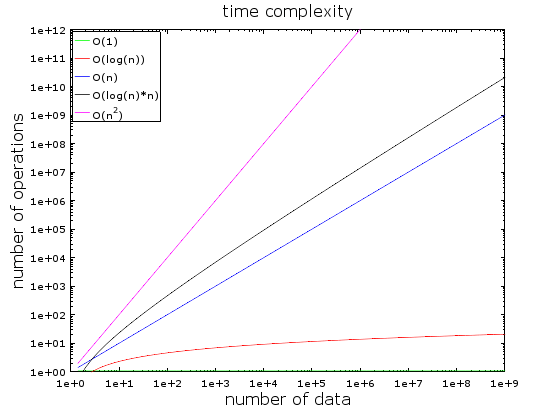## O(n log n) isn’t bad

Posted on Thu 19 October 2017 in Programming • Tagged with algorithms, complexity, Big OLeave a comment

Most programmers should be familiar with the Big O notation of computational complexity. This is how, in very theoretical terms, we are describing the relative differences in the performance of algorithms.

Excluding the case of constant time complexity (`O(1)`), the vast majority of practical algorithms falls into one of the following classes:

• `O(log n)`
• `O(n)`
• `O(n log n)`
• `O(n²)`

The further down a class is on this list, the worse (less efficient) it gets. What may not be completely obvious, however, is the magnitude of differences.

Let’s have a closer look.

#### The best and the worst

First, it’s pretty easy when it comes to the extreme points. A logarithmic complexity is clearly great, because the number of operations barely even grows as the size of input increases. For `N` of one million, the (natural1) logarithm is equal to about 14. For one trillion — million times more — `log n` is only 27!

Such amazing scalability is one of the reasons why databases, for example, can execute queries extremely efficiently even for millions or billions of records.

On the other end, an algorithm that has quadratic complexity will only do well for very small datasets. It can still be useful in practice, especially as a small-input optimization of some larger procedure2, or because of some other desirable properties (like good parallelizability).

Outside of those carefully selected cases, however, the computational requirements of `O(n²)` for any large dataset are usually too great.

#### Middle ground

As for the remaining two classes, the linear one (`O(n)`) is probably the easiest to reason about.

In a linear algorithm, the number of operations increases steadily along with the size of input.
For thousand elements, you need roughly a thousand steps (times a constant factor).
For a million, there will be a million operations necessary.

Thus, by itself, the linear scaling doesn’t get any better or worse when data gets bigger3. In many cases, it means there is nothing to be exploited in the structure of input set that could make the running time any better (compared to e.g. the reliance of logarithmic searches on sorted order). Typically, all the data must be traversed at least once in its entirety.

All in all, it can be a decent time complexity, but it’s nothing to write home about.

#### A function has no name

What about `O(n log n)`, then? It falls between the linear and the quadratic, which suggests that it’s somewhere half-way between mediocre and awful. We don’t even have a widely used word for it, meaning it is probably not even that important.

Both of those suppositions are wrong.

First, `O(n log n)` isn’t even remotely close to the “median” (whatever that means) of `O(n)` and `O(n²)`. In reality, its asymptotic rate of growth places it very close to the former. You can see this pretty clearly by looking at the following plot:Source

The gap between `O(n)` and `O(n log n)` barely even widens, even as the values on vertical axis increase to the limits of practicality.

Indeed, the `log n` part of the function grows slowly enough that, for many practical purposes, it can be considered a large “constant” in the complexity formula. Some complicated algorithm that’s technically linear may therefore be a worse choice than a simpler solution with `O(n log n)` scaling.

#### Sorting it out

What are the typical situations where `O(n log n)` arises in practice? Very often, it has to do with establishing some kind of ordering of the input which includes at least one of the following:

• a wholesale sorting of it (using pairwise comparison)
• repeated queries for the current maximum or minimum (via a priority queue)

Considering that many practical algoithms — from pathfinding to compression — utilize some form of sorting or sorted data structures, it makes `O(n log n)` quite an important complexity class.

1. Natural logarithm has a base of e = 2.71828183… The exact choice of logarithm base doesn’t matter for asymptotic complexity, because it changes only the constant coefficient in the `O(f(n))` function.

2. A widely used example is Timsort which switches from merge sort (`O(log n)`) to insertion sort (`O(n²)`) when the array slice is short enough to warrant it.

3. In reality, practical factors like memory/cache size, OS scheduling behavior, and a myriad of other things can make the actual running time scale sublinearly beyond a certain point.